# Section G.3. MULTISECTION COMPLEX FSF PHASE

### G 3 MULTISECTION COMPLEX FSF PHASE

This appendix shows how the (-1)k factors arise in Eq. (7-13) for an even-N multisection linear-phase complex FSF. Substituting the positive-frequency, 0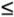k(N/2)–1, |H(k)|ejf(k) gain factors, with f(k) phase values from Eq. (7-11), into Eq. (7-10) gives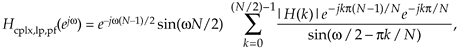where the subscript "pf" means positive frequency. Focusing only on the numerator inside the summation in Eq. (G-16), it is

Equation G-17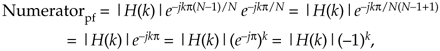showing how the (–1)k factors occur within the first summation of Eq. (7-13). Next we substitute the negative-frequency |H(k)|ejf(k) gain factors, (N/2)+1kN–1, with f(k) phase values from Eq. (7-11''), into Eq. (7-10) giving

Equation G-18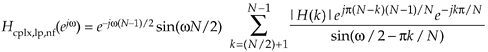where the subscript "nf" means negative frequency. Again, looking only at the numerator inside the summation in Eq. (G-18), it is

Equation G-19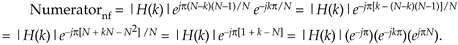That ejpN factor in Eq. (G-19) is equal to 1 when N is even, so we write

Equation G-20establishing both the negative sign before, and the (–1)k factor within, the second summation of Eq. (7-13). To account for the single-section for the k = N/2 term (this is the Nyquist, ors/2, frequency, where w = p) we plug the |H(N/2)|ej0 gain factor, and k = N/2, into Eq. (7-8) giving

Equation G-21URL http://proquest.safaribooksonline.com/0131089897/app07lev1sec3Amazon